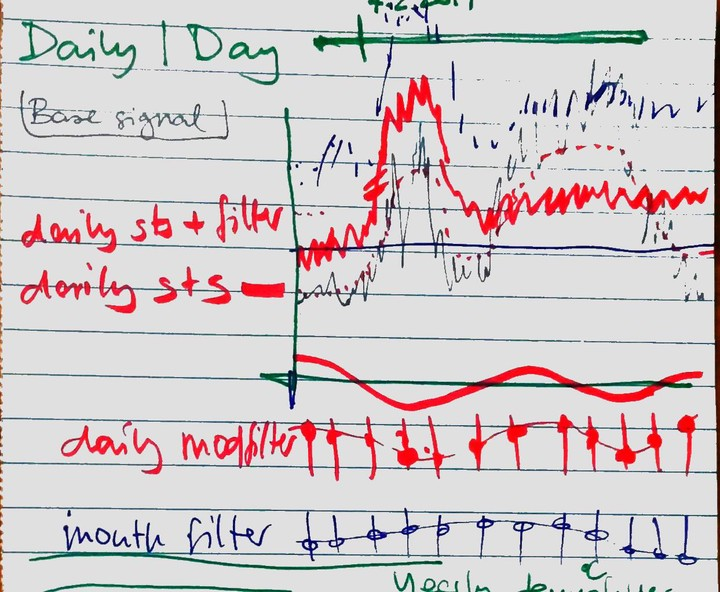# Acoustic equalizer for energy timeseries

Demand and supply timeseries shaping for varying time and semantic filtes# Signal Equalizer

With

• yearly hourly timeseries

equalized_signal

• hat
• base signal or base signal query
• filter array (hourly, daily, monthly, yearly factors [-1;1]
• filter boost series = base signal * filter
• description?
• normalize method
• normalize for timeframe / filter > seems to be the same as regular normalize
• hat repr
• entsteht durch * recalculates (controls) > no

tests

classDiagram
class utils{
normalize(<timeseries>): <timeseries>
mean(<timeseries>): <timeseries>
}

classDiagram
class Equalizer {
<<timeseries>> base signal / base signal query
<<timeseries>> filter boost series = base signal * filter
dict<Filter> filter (hourly, daily, monthly, yearly factors [-1;1]
<<timeseries>> filter boost series = base signal * filter
__repr__(): <timeseries>
}

classDiagram
class NormalizedSignal {
<<timeseries>>
apply_filter(Filter): EqualizedSignal
}

classDiagram
class Filter {
<<timeseries>>
cat timeframe
}


new signal = equalized_signal(base_signal[s], hooks)

• base signal * applicable filters (default 1)

signal_partial =

• for each carrier
• for each filter
• multiply (scale)

store filters

• hour_of_the_day_weights for each carrier (daily)
• day_of_the_week? weights
• month of the year weights (Seasonal)

vizualize filters / partials

• normalized
• as stacked area/bar plot
• base year factor
• seasonal factor
• weekday factor
• hourly factor
• as carpetplots

visualize sts_signal

• with structured time series as sts:
• for average, min, max daily sts signal
• for average, min max yearly sts signal
• as carpetplot

visualize example_signal

• winter WD, WE, summer, WD, WE als nebeneinander lines dropdown?
• sunny / cloudy
• windy / calm
• hot / cold

control filters

• slider for each anchorpoint
• 12 * 2h, day of year slider
• 7 * 1 day, 2 * holiday, month slider
• 12 * Month
• year

dashboard

• for each timeframe
• visualize examples & filter partials
• visualize controls##### Simon Schneider
###### Team lead Livable Positive Energy Districts (KolPEQ)

My research interests include energy analytics, energy information design, energy system theory and positive energy districts.